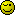# Math.random

``````function RandNum(min, max){
return Math.floor(Math.random() * (min - max) )

}

Lucky = RandNum(1, 6)

console.log(Lucky)
``````

In console, it goes in negatives from -1 to -5, why?

Because min-max is -5 and Math.floor rounds to the nearest integer less than the real number.

1 Like

If you need positive numbers, reverse your formula to (max - min)

If you are using ES6, you can shorten the code to:

``````function RandNum(min, max){
return ~~(Math.random() * (max - min) )

}
``````

A very good example why shorten a code is often not a good solution in case of readable code2 Likes

I must agree, that hacks such as ~~ are far less preferable to using techniques such as Math.floor() which almost everyone can understand at a glance.

We are not desperate for the few extra characters afforded to us by ~~. Our keyboards and fingers can take the strainI’m in agreement here with the use of obfuscation — there’s irony in that word I am sure.

That said it’s good to know about these things. The `~~` is a double bit wise not operation.

As I say I am with the others on this. Would have jumped on it some years ago, but would favour readability nowedit: I believe what you are looking for is this

``````function RandNum(min, max){
return Math.floor(Math.random() * (max - min + 1) + min)
}
``````

Now how readable is that3 Likes

Too advance for me, but I will get there in a decade or maybe 3

1 Like

and also, why does it go in negatives when doing my form? can you explain?

This is simple mathematic.

Min is lower then max. So min - max is a negativ number, if you multiply a positiv random number with a negativ number the result is negative.

1 Like

This topic was automatically closed 91 days after the last reply. New replies are no longer allowed.